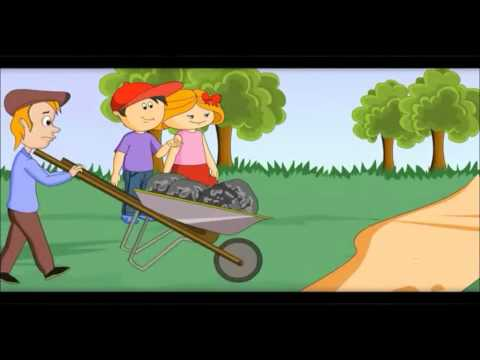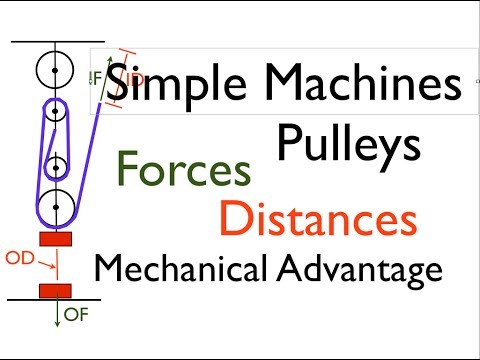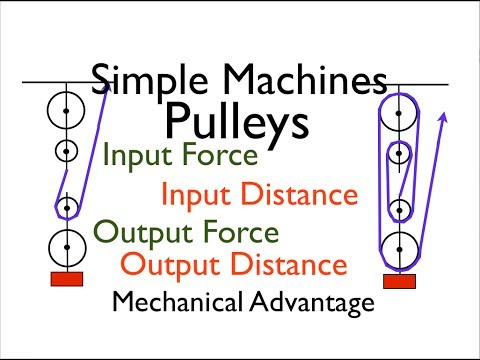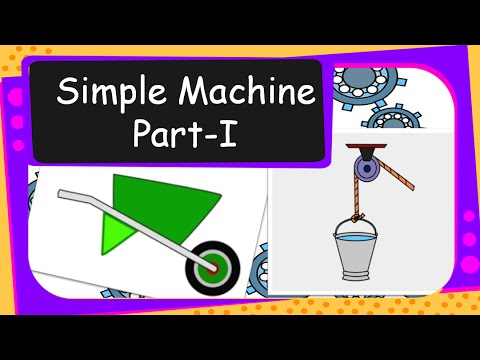## Simple Machine

Subject: Science#### Overview

Simple machines are those in which effort is applied at a more convenient point in a more convenient direction. This note contains information about various types of simple machine with its example, principal of simple machine.

##### Simple Machine

We use different kinds of machines and tools in our daily life. Simple machines are those instruments in which effort is applied at a more convenient point in a more convenient direction. The work done on a machine is called input and the work done by the machine is called output. Input is the product of force and distance through which force is applied and output is the product of load and distance through which load is moved. A simple machine is a device, which makes our work easier by

1. increasing the force applied
2. changing the direction of force applied
3. transferring the force from one place to another
4. increasing the speed of a forceAll machines work on the principle that when the effort is smaller than the load, it has to move a greater distance than the load in order to lift it up. There are six types of simple machines, they are:

1. Lever
2. Pulley
3. Wheel and axle
4. Inclined plane
5. Screw
6. Wedge

#### Principle of a simple machine

The principle of machine is Input work = Output work

Or, E x E. d = L x L. d

Where, E = Effort

E. d = Effort distance

##### Things to remember
• A simple machine is a device, which makes our work easier by

1. increasing the force applied
2. changing the direction of force applied
3. transferring the force from one place to another
4. increasing the speed of a force
• Types of simple machines are:

1. Lever
2. Pulley
3. Wheel and axle
4. Inclined plane
5. screw
6. Wedge
• The principal of machine is Input work = Output work

• It includes every relationship which established among the people.
• There can be more than one community in a society. Community smaller than society.
• It is a network of social relationships which cannot see or touched.
• common interests and common objectives are not necessary for society.
##### Videos for Simple Machine##### Lever -introduction to 3 types - Simple Machines lesson for kids by www.makemegenius.com##### Part 1 - Simple Machines, Pulleys; Forces, Distances and MA##### Part 3 - Simple Machines, Pulleys; Distances, Forces and MA##### Science - Simple machine (Inclined plane, Wheel and axle and Pulley)

A simple machine makes the work easier and faster by:

• Increasing the force applied
• Changing the direction of force applied
• Transferring the force from one place to another
• Increasing the speed of a force

A machine is a device when force is applied to it, it makes our work easier, changes the direction of force and enhance the speed of the work.

The two types of simple machines are lever and Pulley.

There are six types of simple machines, they are:

1. Lever
2. Pulley
3. Wheel and axle
4. Inclined plane
5. Screw
6. Wedge

The principal of machine is

Input work = Output work

Or, E x E. d = L x L. d

Where, E = Effort

E. d = Effort distance